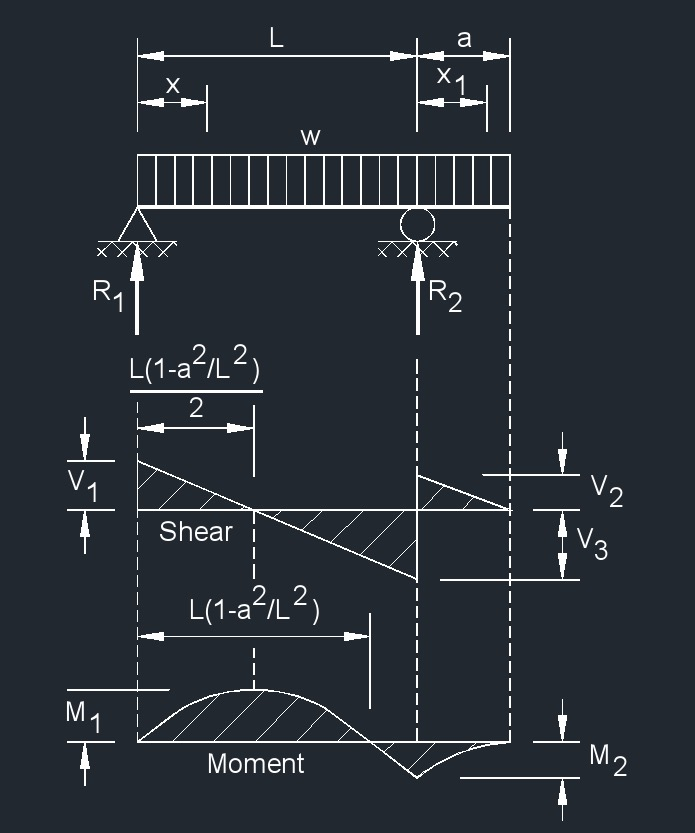# Overhanging Beam - Uniformly Distributed Load

Written by Jerry Ratzlaff on . Posted in Structural

##formulas that use Overhanging Beam - Uniformly Distributed Load

 $$\large{ R_1 = V_1 = \frac{w}{2L} \left( L^2 - a^2 \right) }$$ $$\large{ R_2 = V_2 + V_3 = \frac{ w }{2L} \left( L + a \right)^2 }$$ $$\large{ V_2 = wa }$$ $$\large{ V_3 = \frac{ w }{2L} \left( L^2 + a^2 \right) }$$ $$\large{ V_x \; }$$  (between supports)    $$\large{ = R_1 - wx }$$ $$\large{ V_{x_1} \; }$$  (for overhang)    $$\large{ = w \left( a - x_1 \right) }$$ $$\large{ M_x \; }$$  (between supports)    $$\large{ = \frac{ wx }{2L} \left( L^2 - a^2 - xL \right) }$$ $$\large{ M_{x_1} \; }$$  (overhang)    $$\large{ = \frac{ w }{2} \left( a - x_1 \right)^2 }$$ $$\large{ M_2 \; }$$  at   $$\large{ \left( R_2 \right) = \frac{ wa^2 }{2} }$$ $$\large{ M_1 \; }$$  at   $$\large{ \left[ x = \frac{L}{2} \left( 1 - \frac{a^2}{L^2} \right) \right] = \frac{ w }{8 L^2} \left( L + a \right)^2 \left( L - a \right)^2 }$$ $$\large{ \Delta_x \; }$$  (between supports)   $$\large{ = \frac { w x} { 24 \lambda I L} \left( L^4 - 2L^2x^2 + Lx^3 - 2a^2L^2 + 2a^2x^2 \right) }$$ $$\large{ \Delta_{x_1} \; }$$  (for overhang)   $$\large{ = \frac { w x_1} { 24 \lambda I } \left( 4a^2L - L^3 + 6a^2x_1 - 4ax_{1}{^2} + x_{1}{^3} \right) }$$

### Where:

$$\large{ I }$$ = moment of inertia

$$\large{ L }$$ = span length of the bending member

$$\large{ M }$$ = maximum bending moment

$$\large{ R }$$ = reaction load at bearing point

$$\large{ V }$$ = shear force

$$\large{ w }$$ = load per unit length

$$\large{ W }$$ = total load from a uniform distribution

$$\large{ x }$$ = horizontal distance from reaction to point on beam

$$\large{ \lambda }$$   (Greek symbol lambda) = modulus of elasticity

$$\large{ \Delta }$$ = deflection or deformation